Question

Using Matlab. I need the matlab code for:

a. Plot a 1 Hz sine wave

b. Plot the unit rectangle function.

c. Plot the unit triangle function

Show each over a plotting interval -5 sec to 5 sec.

1)

To Plot 1Hz sine wave:

-------------------------------------------------------------------------------------------------------------------------

Fs = 10000; % samples per second

dt = 1/Fs; % seconds per sample

StopTime = 5; % seconds

t = (-5:dt:StopTime-dt)'; % seconds

%%Sine wave:

Fc = 1; % hertz

x = cos(2*pi*Fc*t);

% Plot the signal versus time:

figure;

plot(t,x);

xlabel('time (in seconds)');

title('Signal versus Time');

--------------------------------------------------------------------------------------------------------------------------------

2)

Plotting unit Rectangle function:

--------------------------------------------------------------------------------------------------------------------------------

rectangle=@(x,a) ones(1,numel(x)).*(abs(x)<a/2) % a is the width of the pulse

x=-5:0.001:5

y=rectangle(x,2)

plot(x,y)

----------------------------------------------------------------------------------------------------------------------------------

3)

Plotting unit Triangle function:

-----------------------------------------------------------------------------------------------------------------------------------

t = -5:0.005:5;

g_t = 0;

g_a = sin(2*pi*t)+(0.5*cos(6*pi*t))+(0.25*cos(18*pi*t)); %given

B = 10;

for n = 1:20

h = 2*B*t-n;

g_t = g_t + (n/(2*B)).*g_a.*(1-abs(2*B*t-n));

end

figure(1);

hold on;

box on;

plot(t,g_t,'r');

plot(t,g_a,'b');

ylabel('amplitude g(t)');

xlabel('time t');

title('sync waveform reconstruction');

axis([0 1 -2 2]);

----------------------------------------------------------------------------------------------------------------------------------------------------------

#### Earn Coins

Coins can be redeemed for fabulous gifts.

Similar Homework Help Questions
• ### Please answer this question using Matlab: Write a matlab function to generate and plot a sine...

Please answer this question using Matlab: Write a matlab function to generate and plot a sine wave at a specified frequency having at least 10 cycles, for this case the amplitude will represent voltage. Your sine wave should have a positive going zero crossing at T=0. Make sure your plot has at least 25 samples per cycle. Make sure the plot is with respect to time. Your function needs to return your generated samples and time vector. The frequency will...

• ### Need help with matlab code. If writing code out please make it where i can understand...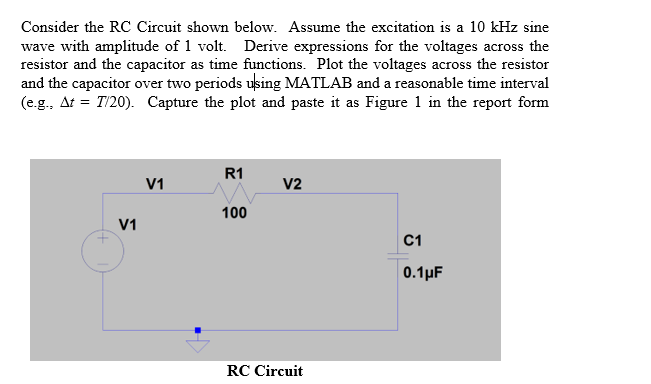Need help with matlab code. If writing code out please make it where i can understand and read it. I would prefer to see it in matlab form. Thanks Consider the RC Circuit shown below. Assume the excitation is a 10 kHz sine wave with amplitude of 1 volt. Derive expressions for the voltages across the resistor and the capacitor as time functions. Plot the voltages across the resistor and the capacitor over two periods using MATLAB and a reasonable...

• ### I need help with Matlab code. Thank you Consider the RC Circuit shown below. Assume the...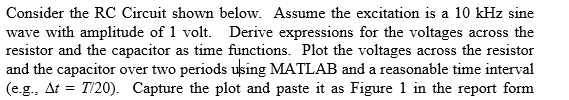I need help with Matlab code. Thank you Consider the RC Circuit shown below. Assume the excitation is a 10 kHz sine wave with amplitude of 1 volt. Derive expressions for the voltages across the resistor and the capacitor as time functions. Plot the voltages across the resistor and the capacitor over two periods using MATLAB and a reasonable time interval (eg, = T 20) Capture the plot and paste it as Figure 1 in the report form

• ### 6) Consider the below wave form when a-1 Hz (2n rad/sec) and b-0.9a F(t) sin(at)+sin(bt) I. Calcu...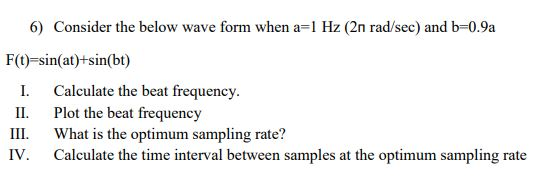Please help, the plotting can be done in excel or matlab 6) Consider the below wave form when a-1 Hz (2n rad/sec) and b-0.9a F(t) sin(at)+sin(bt) I. Calculate the beat frequency. II. Plot the beat frequency II. What is the optimum sampling rate? IV. Calculate the time interval between samples at the optimum sampling rate 6) Consider the below wave form when a-1 Hz (2n rad/sec) and b-0.9a F(t) sin(at)+sin(bt) I. Calculate the beat frequency. II. Plot the beat frequency...

• ### Please include your matlab code and plot, as well as the derivation of the expressions for...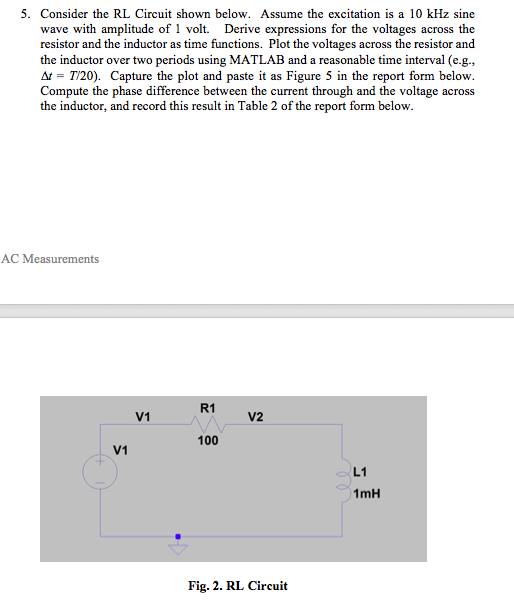Please include your matlab code and plot, as well as the derivation of the expressions for voltages across both the resistor and inductor themselves. Really need help understanding this! 5. Consider the RL Circuit shown below. Assume the excitation is a 10 kHz sine wave with amplitude of 1 volt. Derive expressions for the voltages across the resistor and the inductor as time functions. Plot the voltages across the resistor and the inductor over two periods using MATLAB and a...

• ### MATLAB question I need the code exactly as you would write it in MATLAB. thank you...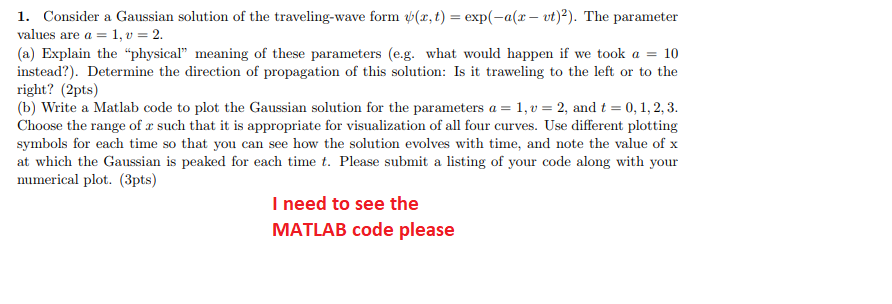MATLAB question I need the code exactly as you would write it in MATLAB. thank you 1. Consider a Gaussian solution of the traveling-wave form ψ(z,t)-exp(-a(z-vt)2). The parameter values are a 1, 2. (a) Explain the physical meaning of these parameters (eg. what would happen if we took a = 10 instead?) Determine the direction of propagation of this solution: Is it traweling to the left or to the right? (2pts) (b) Write a Matlab code to plot the Gaussian...

• ### (B) Implement Matlab code for each part as described below: i) Define the following signal in tim...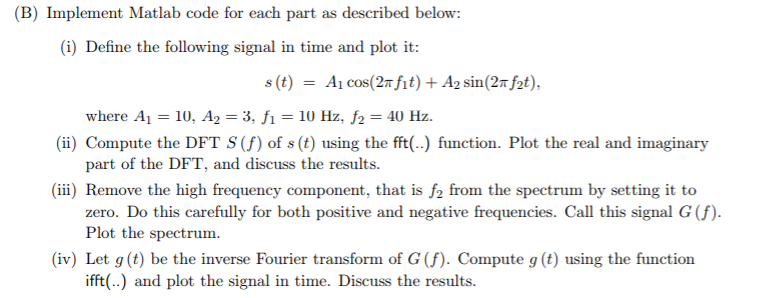Using MATLAB, Please read carefully, EXPLAIN CODE AND ANSWERS, DISCUSS RESULTS, I NEED EVERY PART (B) Implement Matlab code for each part as described below: i) Define the following signal in time and plot it where Ai 10, A2-3, fi-10 Hz, f2-40 Hz. part of the DFT, and discuss the results. zero. Do this carefully for both positive and negative frequencies. Call this signal G (f). ii) Compute the DFT S(f) of s (t) using the fft() function. Plot the...

• ### by using Matlab R20 16a I need the code and the graph * Write a program...

by using Matlab R20 16a I need the code and the graph * Write a program to plot the derivative of function ?=?.?^−?^?

• ### Using MATLAB, duplicate to the two graphs shown in the figure below Requirements 1. Show the MATLAB code used to plot both functions 5cos (nt-ng) (V) 5 e-"X cos (2nft-2nx/A) (V) a. y b- Use the f...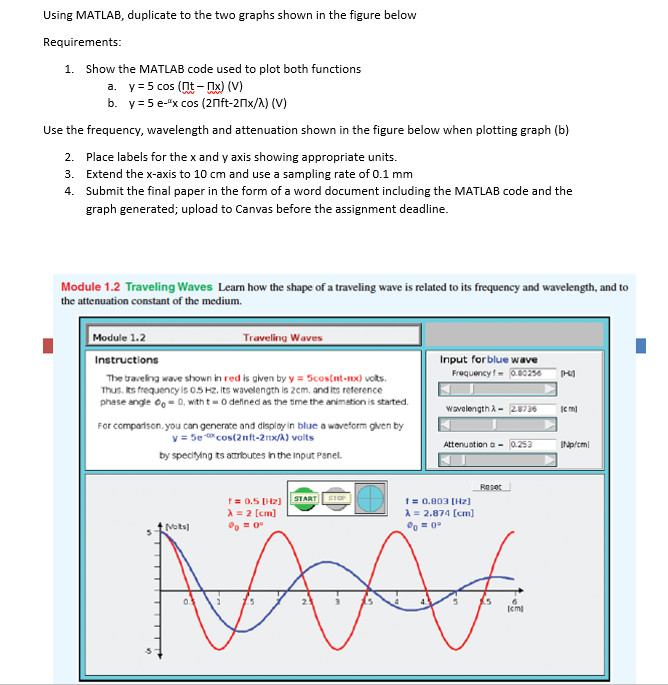Using MATLAB, duplicate to the two graphs shown in the figure below Requirements 1. Show the MATLAB code used to plot both functions 5cos (nt-ng) (V) 5 e-"X cos (2nft-2nx/A) (V) a. y b- Use the frequency, wavelength and attenuation shown in the figure below when plotting graph (b) 2. 3. 4. Place labels for the x and y axis showing appropriate units Extend the x-axis to 10 cm and use a sampling rate of 0.1 mm Submit the final...

• ### This problem needs to be solved using MATLAB! I need help with this homework. I will...

This problem needs to be solved using MATLAB! I need help with this homework. I will give 100% rating to the answer that is successfully executed and completed. Please provide screenshots of both the MATLAB code and the plots. Problem 4: sketch the signal g(t) = t2 and find the exponential Fourier series to represent g(t) over the interval [-1,1] (Find Dn' s). Plot Dn for n = -20:20. (use stem function in MATLAB and provide your code as well)...